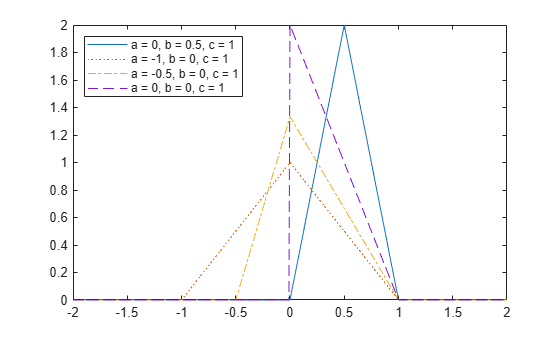## Triangular Distribution

### Overview

The triangular distribution provides a simplistic representation of the probability distribution when limited sample data is available. Its parameters are the minimum, maximum, and peak of the data. Common applications include business and economic simulations, project management planning, natural phenomena modeling, and audio dithering.

### Parameters

The triangular distribution uses the following parameters.

ParameterDescriptionConstraints
`a`Lower limit$a\le b$
`b`Peak location$a\le b\le c$
`c`Upper limit$c\ge b$

#### Parameter Estimation

Typically, you estimate triangular distribution parameters using subjectively reasonable values based on the sample data. You can estimate the lower and upper limit parameters a and c using the minimum and maximum values of the sample data, respectively. You can estimate the peak location parameter b using the sample mean, median, mode, or any other subjectively reasonable estimate of the population mode.

### Probability Density Function

The probability density function (pdf) of the triangular distribution is

`$f\left(x|a,b,c\right)=\left\{\begin{array}{c}\frac{2\left(x-a\right)}{\left(c-a\right)\left(b-a\right)}\text{ };\text{ }a\le x\le b\\ \frac{2\left(c-x\right)}{\left(c-a\right)\left(c-b\right)}\text{ };\text{ }bc\end{array}\text{ }.$`

This plot shows how changing the value of the parameters a, b, and c alters the shape of the pdf.

```% Create four distribution objects with different parameters pd1 = makedist('Triangular'); pd2 = makedist('Triangular','a',-1,'b',0,'c',1); pd3 = makedist('Triangular','a',-.5,'b',0,'c',1); pd4 = makedist('Triangular','a',0,'b',0,'c',1); % Compute the pdfs x = -2:.01:2; pdf1 = pdf(pd1,x); pdf2 = pdf(pd2,x); pdf3 = pdf(pd3,x); pdf4 = pdf(pd4,x); % Plot the pdfs figure; plot(x,pdf1,'r','LineWidth',2) hold on; plot(x,pdf2,'k:','LineWidth',2); plot(x,pdf3,'b-.','LineWidth',2); plot(x,pdf4,'g--','LineWidth',2); legend({'a = 0, b = 0.5, c = 1','a = -1, b = 0, c = 1',... 'a = -0.5, b = 0, c = 1','a = 0, b = 0, c = 1'},'Location','NW'); hold off;```As the distance between a and c increases, the density at any particular value within the distribution boundaries decreases. Because the density function integrates to 1, the height of the pdf plot decreases as its width increases. The location of the peak parameter b determines whether the pdf skews right or left, or if it is symmetrical.

### Cumulative Distribution Function

The cumulative distribution function (cdf) of the triangular distribution is

`$F\left(x|a,b,c\right)=\left\{\begin{array}{c}\text{ }\text{ }\text{ }\text{ }\text{ }0\text{ },\text{ }xc\end{array}\text{ }.$`

This plot shows how changing the value of the parameters a, b, and c alters the shape of the cdf.

```% Create four distribution objects with different parameters pd1 = makedist('Triangular'); pd2 = makedist('Triangular','a',-1,'b',0,'c',1); pd3 = makedist('Triangular','a',-.5,'b',0,'c',1); pd4 = makedist('Triangular','a',0,'b',0,'c',1); % Compute the cdfs x = -1.2:.01:1.2; cdf1 = cdf(pd1,x); cdf2 = cdf(pd2,x); cdf3 = cdf(pd3,x); cdf4 = cdf(pd4,x); % Plot the cdfs figure; plot(x,cdf1,'r','LineWidth',2) xlim([-1.2 1.2]); ylim([0 1.1]);hold on; plot(x,cdf2,'k:','LineWidth',2); plot(x,cdf3,'b-.','LineWidth',2); plot(x,cdf4,'g--','LineWidth',2); legend({'a = 0, b = 0.5, c = 1','a = -1, b = 0, c = 1',... 'a = -0.5, b = 0, c = 1','a = 0, b = 0, c = 1'},'Location','NW'); hold off;```### Descriptive Statistics

The mean and variance of the triangular distribution are related to the parameters a, b, and c.

The mean is

`$\text{mean}=\left(\frac{a+b+c}{3}\right)\text{\hspace{0.17em}}.$`

The variance is

`$\mathrm{var}=\left(\frac{{a}^{2}+{b}^{2}+{c}^{2}-ab-ac-bc}{18}\right)\text{\hspace{0.17em}}.$`